Congruent Parts
Congruency Statements
Theorems/Postulates
Isosceles Triangles
Equilateral Triangles
Random Triangles
100

Write the 3 congruent angle pairs.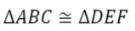100

Write the congruency statement: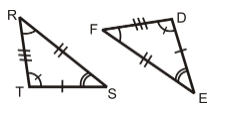100

State how these triangles are congruent.100

Find x.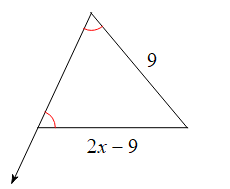100

Find x.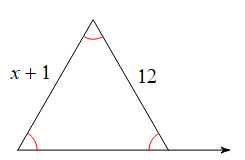100

Classify by side and angle.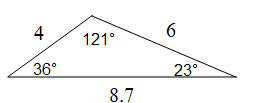200

Write the 3 congruent pairs of sides.200

Write the congruency statement: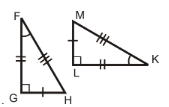200

State how these triangles are congruent.200

Find x.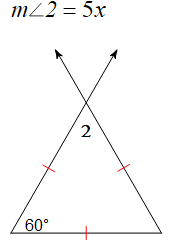200

Find x.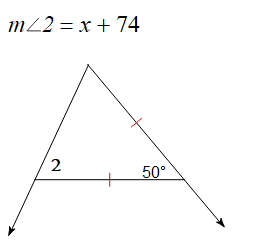200

Find x.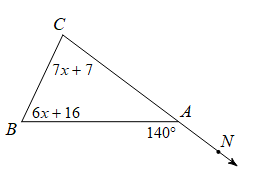300

Write the 3 congruent pairs of sides.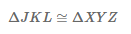300

Write the congruency statement: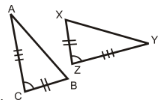300

State how these triangles are congruent.

300

Find x.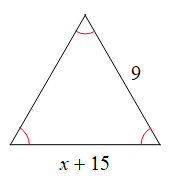300

Find x.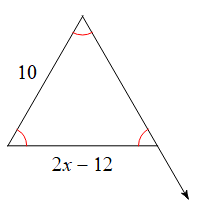300

Find the measurement of angle A.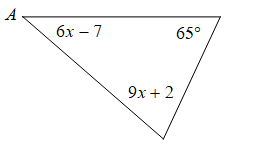400

Write the 3 congruent pairs of angles.400

Write the congruency statement:400

State how these triangles are congruent.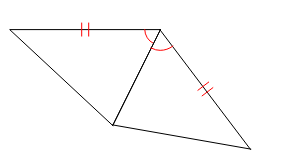400

Find x.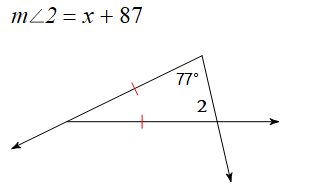400

Find x.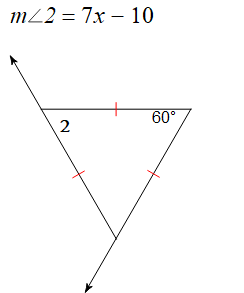400

Find the measurement of angle A.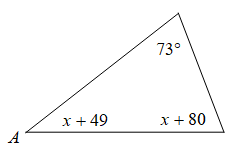500

Write the 6 congruent pairs.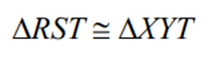500

Write the congruency statement: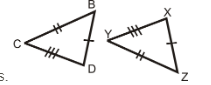500

State how these triangles are congruent.500

Find x.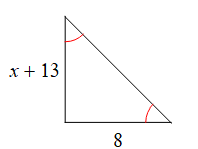500

Find x.500

Find x.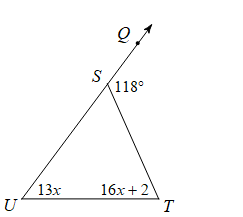600

Write the 6 congruent pairs.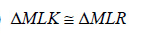600

Write the congruency statement: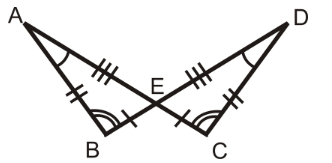600

State how these triangles are congruent.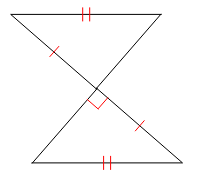600

Find x.600

Find x.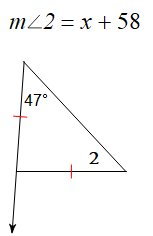600

Classify by side and angle.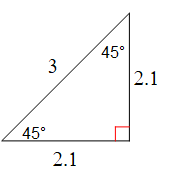Click to zoom